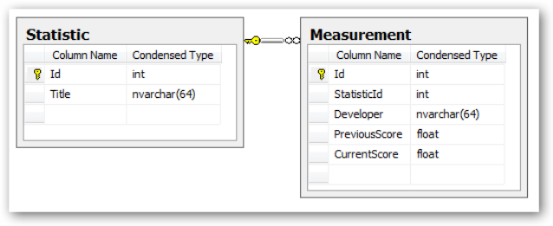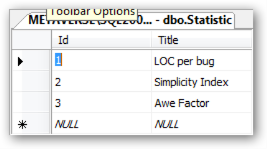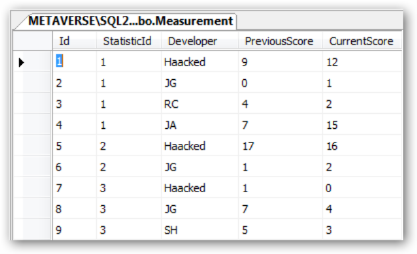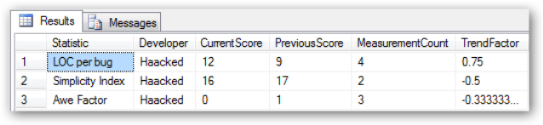I’m not one to post a lot of quizzes on my blog. Let’s face it, while we may create altruistic reasons for posting quizzes such as:

1. It’s an interesting problem I thought up
2. It’s an interesting bug I ran into

we all know the real reasons for posting a quiz.

1. It serves as blog filler.
2. It’s a way to show off how smart the blogger is.

With that in mind, let me humbly present my latest SQL Quiz, which is something I ran into at work recently, and will not show off any smarts whatsoever.

The circumstances of this problem have been dramatically changed and simplified to both protect the guilty and save me from a lot of typing.

In this application, we have two tables. One contains a lookup list of various statistics. The second is a larger table of measurements for each of the statistics.

The following screenshot shows the data model.The following screenshot shows the list of contrived statistics.What we see above are the following:

1. LOC per bug - Lines of code per bug.
2. Simplicity Index - some magical number that purports to measure simplicity.
3. Awe Factor - The awe factor for the source code.

For each of these statistics, the larger, the better.

The following is a view of the Measurement table.Each measurement has the previous score and current score (this is a denormalized version of the actual tables for the purposes of demonstration).

I needed to write a query that would show each of the stats for a given developer as well as a Trend Factor. The Trend Factor tells you whether or not the statistic is trending positive or negative, where positive is better and negative is worse.Here is my first cut at the stored procedure. It’s pretty straightforward. In order to make the important part of the query as clear as possible, I used a Common Table Expression to make sure the count of measurements for each statistic can be referenced as if it were a column.

``````CREATE PROC [dbo].[Statistics_GetForDeveloper](
@Developer nvarchar(64)
)
AS
WITH MeasurementCount(StatisticId, MeasurementCount) AS
(
SELECT s.Id
,MeasurementCount = COUNT(1)
FROM Statistic s
LEFT OUTER JOIN Measurement m ON m.StatisticId = s.Id
GROUP BY s.Id
)
SELECT
Statistic = s.Title
, Developer
, CurrentScore
, PreviousScore
, mc.MeasurementCount
, TrendFactor = (CurrentScore - PreviousScore)/mc.MeasurementCount
FROM Statistic s
INNER JOIN MeasurementCount mc ON mc.StatisticId = s.Id
LEFT OUTER JOIN Measurement m ON m.StatisticID = s.Id
WHERE Developer = @Developer
GO
``````

I bolded the relevant part of the query. We calculate the TrendFactor by taking the current score, subtracting the previous score, and then dividing the difference by the number of measurements for that particular statistic. This tells us how that statistic is trending.

In this application, I am going to present an up arrow for trend factors larger than 0.1, a down arrow for trend factors less than -0.1, and a flat line for anything in between. A trend factor going upward is always considered a “good thing”.

### The Challenge

This works for now because for each statistic, a larger value is considered better. But we need to add a new statistic, Deaths per LOC, which measures the number of deaths per line of code (gruesome, yes. But whoever said this industry is all roses and rainbows?). For this statistic, an upward trend is a “bad thing”.

Therefore, if the current score is larger than the previous score for this statistic, we would want the TrendFactor to be negative. Not only that, we may want to add more statistics in the future. Some for which larger values are better. And some for which smaller values are better.

So here is the quiz question. You are allowed to make a schema change to the Statistic table and to the stored procedure. What changes would you make to fulfill the requirements?

Bonus points, can you fulfill the requirements without using a `CASE` statement in the stored procedure?

Here is a SQL script that willl setup the tables and initial stab at the stored procedure for you. The script requires SQL Server 2005 or SQL Server Express 2005.